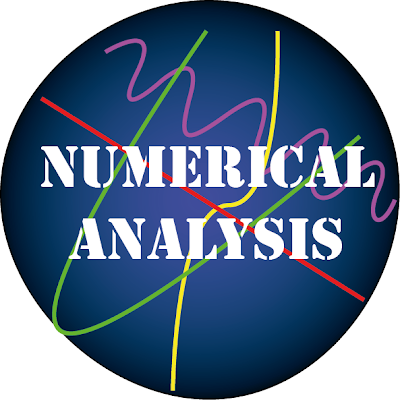# Seminar on the numerical analysis at the college of scienceThe department of mathematics, in collaboration with the continuing education unit at the college of science organized a seminar on the “Numerical Analysis” delivered by Dr. Dalia Khaled and Assist. Lecturer Alaa Walid to identify one of the important fields of mathematics which combines analysis with computer calculations in order to find solutions to some issues and problems that cannot be solved by analytical mathematics and where the results can be approximate.

This event was attended by a number of students, professors and those concerned in mathematics, statistics and computer science where the two lecturers explained that the numerical analysis is the study of algorithms that use numerical approximation for the problems of mathematical analysis, including how to find a possible outcome or an approximate solution to the numerical analysis, in the sense that gives an error rate that leads to reach at the real solution for any calculation. So the task of the numerical analysis is to correct mathematical error through different types of analysis.